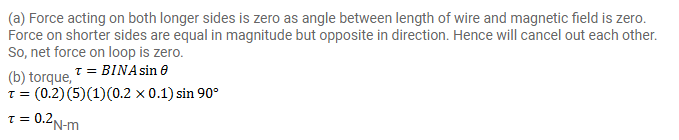# A rectangular loop of sides 20cm and 10cm carries a current of 5.0A.

Question:

A rectangular loop of sides $20 \mathrm{~cm}$ and $10 \mathrm{~cm}$ carries a current of $5.0 \mathrm{~A}$. A uniform magnetic field of magnitude $0.20 \mathrm{~T}$ exists in parallel to the longer side of the loop. (a) What is the force acting on the loop? (b) What is the torque acting on the loop?

Solution: